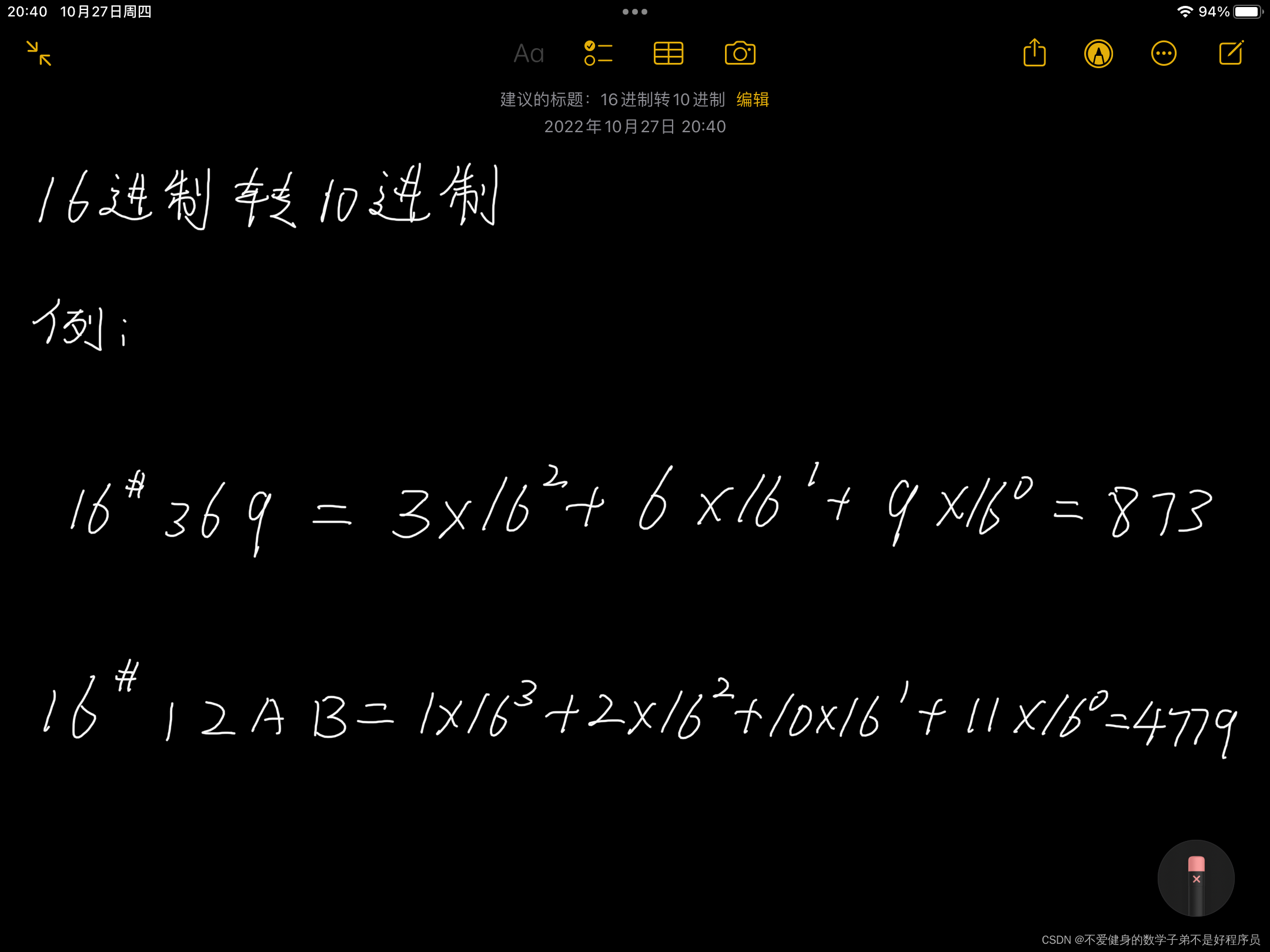# 十六进制转十进制(Java)1.十进制

2.十六进制

# 二、转换方法(思路)

(十进制默认不用写10#)# 三、代码编写

• 先将A(10)、B(11)、C(12)、D(13)、E(14)、F(15)放入HashMap中。
• 循环判断数字部分和字母部分，然后对字母部分判断找出hashmap中对应的value值，最终进行类加得出结果。
``````import java.util.HashMap;
import java.util.Scanner;
import java.util.Set;

/**
* 十六进制转十进制
* 0、1、2、3、4、5、6、7、8、9、A(10)、B(11)、C(12)、D(13)、E(14)、F(15)
*/
public class HexToDec {
public static void main(String[] args) {
int dec = 0;
Scanner sc = new Scanner(System.in);
String hex = sc.nextLine();

//先将A(10)、B(11)、C(12)、D(13)、E(14)、F(15)放入HashMap中
String[] overTen = {"A", "B", "C", "D", "E", "F"};
HashMap<String, Integer> hashMap = new HashMap<>();
for (int i = 0; i < 6; i++) {
hashMap.put(overTen[i], i + 10);
}

//取出hashMap中键的集合
Set<String> keySet = hashMap.keySet();

//循环判断
for (int i = 0; i < hex.length(); i++) {
//判断数字部分
if (hex.charAt(i) >= 48 && hex.charAt(i) <= 57) {
dec += (hex.charAt(i) - 48) * Math.pow(16, hex.length() - 1 - i);
} else {
//判断字母部分
for (String s : keySet) {       //对键的集合进行遍历
if ((hex.charAt(i) + "").equals(s)) {   //判断找出相匹配的键
Integer value = hashMap.get(s);     //根据键找出相对应的值
dec += value * Math.pow(16, hex.length() - 1 - i);  //类加求和
}
}
}
}
System.out.println(hex + "转换为十进制的结果为：" + dec);
}
}
``````THE END